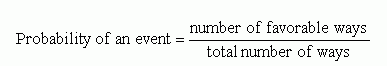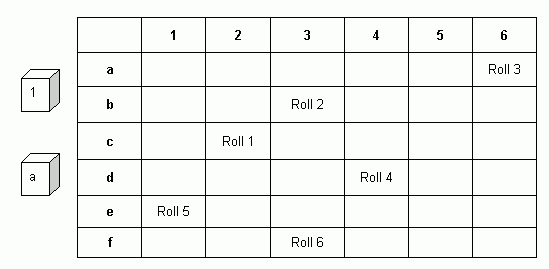Email us to get an instant 20% discount on highly effective K-12 Math & English kwizNET Programs!

#### Online Quiz (WorksheetABCD)

Questions Per Quiz = 2 4 6 8 10

### MEAP Preparation - Grade 7 Mathematics5.1 Probability

 Ratios are statements of comparisons of two quantities. Proportions are equations showing that two ratios are equivalent. Probability Is the chance of an event happening. If a particular outcome can never occur, its probability is 0. If an outcome is certain to occur, its probability is 1. In general, if p is the probability that a specific outcome will occur, values of p fall in the range 0 <= p <= 1. Probability may be expressed as either a decimal, a fraction, or a ratio. Probability examples in everyday life include weather prediction, board games, sports statistics, etc. Examples: What are the chances that the sun will shine this month? (likely) What are the chances that you, a 11 year old boy, will be driving a car today? (unlikely) What are the chances of tossing heads or tails on a coin? (equally likely)Coin: 2 sides The probability of rolling heads is 1/2 The probability of rolling tails is 1/2 Cube: 6 sides Take a number cube and label the sides 1 - 6. The probability of rolling a 3 is one outcome out of a total of six possible outcomes, that is 1/6. Similarly, the probability of rolling a 5 is also 1/6. The probability of rolling an odd number (1, 3, 5) = three outcomes out of a total of six possible outcomes = 3/6 = 1/2 Probability of rolling an even number is also same as rolling an odd numbers (3/6 or 1/2). To help you understand or learn about finding the probability of two or more events, make a letter cube using one of your number cubes (for example, 1 equals the letter a, 2 equals the letter b, 3 equals the letter c, and so on). Roll one cube and one letter cube.Probability of two or more events: first find the probability of each event and then multiply the two probabilities to find the probability of both events occurring together. Example: Rolling a 4 and letter c has a probability of 1/6 (rolling a 4) x 1/6 (rolling a letter c) = 1/36. The probability would be the same for rolling a 2 and rolling a letter e. To find the probability of the above two events, multiply 1/36 x 1/36 which equals 1/1296 Directions: Solve the following problems. Show your work on a sheet of paper. Also write at least 5 examples of your own.
 Q 1: What is the probability of getting a number more than 3 if you roll a dice. 1/411/21/3 Q 2: What is the probability of getting tails with a coin?1/21/41/3 Q 3: What is the probability of getting heads with a coin?1/31/21/4 Q 4: If a jar contains 13 red marbles and 7 green marbles, the probability that a marble selected from the jar at random will be green is ______.07/2013/201 Q 5: What is the probability of rolling a 5 in a dice?1/21/61/5 Q 6: What is the probability of two events: rolling a 4 and letter c and rolling a 2 and rolling the letter e?1/661/12961/36 Question 7: This question is available to subscribers only! Question 8: This question is available to subscribers only!